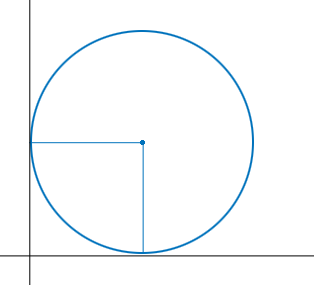SEARCH HOMEMath Central Quandaries & QueriesQuestion from MJ, a student: I'm a College Student taking up Bachelor of Secondary Education on Math Subject. And I'm struggling for my research about Circles. I done solving the said topic particularly on this question: "What are the possible equations of a circle being tangent to a pair of perpendicular lines, having the origin as the Point of Intersection and the C (h, k), where h, k ∈ℤ" But I can't get what would be the process that I must do in order to jive to my idea/goal for that problem. Please check my idea that the numerical coefficients of the equation is equal to the radius of the circle. Thanks in advance! :)Hi,

Did you draw a diagram?The equation of a circle with centre $(h, k)$ and radius $r$ is $(x - h)^2 + (y - k)^2 = r^2 .$ Label $h$, $k$ and $r$ on the diagram. What can you tell about $h, k$ and $r?$ What is the equation of the circle?

PennyMath Central is supported by the University of Regina and The Pacific Institute for the Mathematical Sciences.